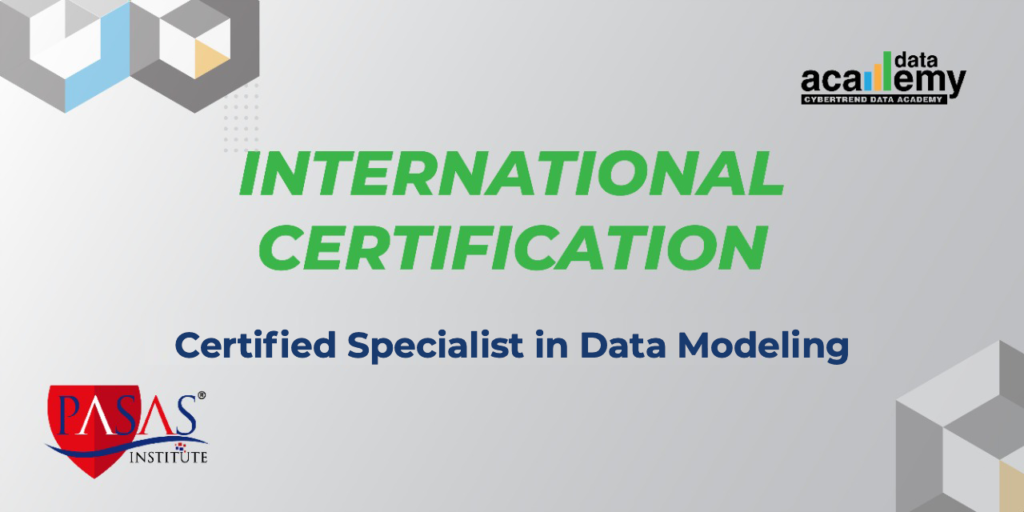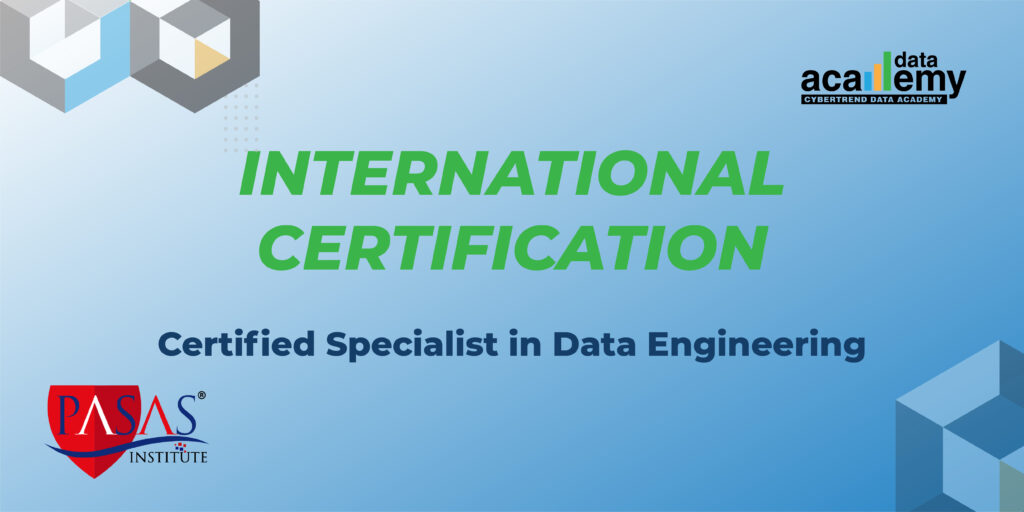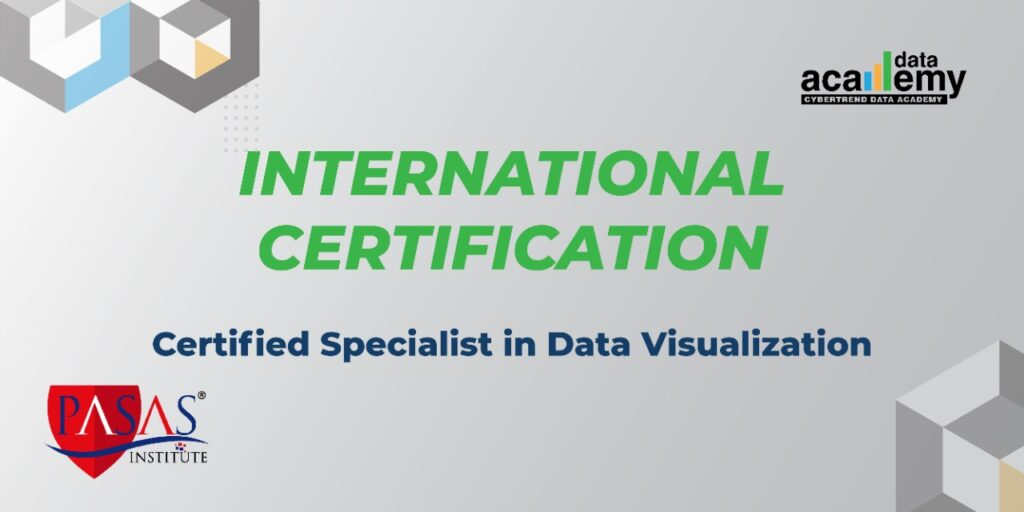1. Introduction to Data Science
2. Introduction to Statistic for Data Science
3. Common statistic used by Data Science
4. Introduction to Data Science Tools (Python)
5. Install and use package
6. Basic Python syntax
7. Connect and explore your data
8. Numpy for data manipulation
9. Graphical and numerical techniques to begin uncovering the structure of data
10. Import, build, and manipulate Data Frame
11. Tidy, rearrange, and structure data
12. Introduction to Data Science Methodology
13. Apply Data Science with Classification (Decision Tree) Model Apply Data Science with Estimation (Regression) Model

## Rp 6.105.0001. Introduction to Data Warehousing
2. Implementation, Operation and Expansion of Data Warehouse
3. Introduction to Data Integration tools
4. Working with files
5. Joining data sources
6. Filtering data
7. Using context variables
8. Error handling
9. Generic schemas
10. Working with databases
11. Creating master Jobs
12. Running a stand-alone Job
13. Documenting a Job

## Rp 6.105.0001. Introduction Data Visualization Concepts
2. Introduction to Data Visualization Tools (Tableau)
3. Connecting to Data
4. Different Chart Types and When to Use Each One
5. Adding Filter, Sort, and Group
6. Work with Time series Data
7. Using Multiple Measure in a View
8. Showing the Relationship Between Numerical Values
9. Mapping Data Geographically
10. Viewing Specific Values
11. Basic Calculations
12. Highlight Data with Reference Line

## Rp 6.105.0001. Understanding Database Concepts
2. Understanding Data Manipulation Language (DML)
3. Understanding Data Definition Language (DDL)
4. Defining Data Types
5. Creating and Using Tables
6. Creating Views
7. Creating Stored Procedures
8. Using Queries to Select Data
9. Using Queries to Insert Data
10. Updating Data and Databases
11. Deleting Data
12. Normalizing a Database
13. Understanding Primary, Foreign, and Composite Keys
14. Understanding Clustered and Non-Clustered Indexes
15. Securing Databases
16. Backing Up and Restoring Databases